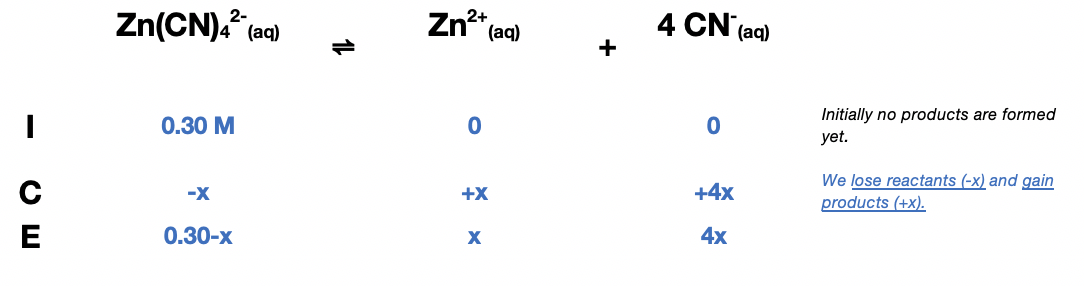# Problem: Calculate the equilibrium concentration of Zn2+ in a 0.30-M solution of Zn(CN)42−.

###### FREE Expert Solution

The equilibrium expression, Kd for the dissociation of the complex Zn(CN)42- is:

**Exponent is equal to the stoichiometric coefficient

First, we need to construct an ICE table for the dissociation of Zn(CN)42-Next, we calculate for x, to determine the equilibrium concentration of Zn2+ and CN-

Kd = 2.1 × 1019

${\mathbf{K}}_{\mathbf{d}}\mathbf{=}\mathbf{2}\mathbf{.}\mathbf{1}\mathbf{×}{\mathbf{10}}^{\mathbf{19}}\mathbf{=}\frac{\mathbf{\left[}{\mathbf{Zn}}^{\mathbf{2}\mathbf{+}}\mathbf{\right]}{\mathbf{\left[}{\mathbf{CN}}^{\mathbf{-}}\mathbf{\right]}}^{\mathbf{4}}}{\mathbf{\left[}\mathbf{Zn}{{\mathbf{\left(}\mathbf{CN}\mathbf{\right)}}_{\mathbf{4}}}^{\mathbf{2}\mathbf{-}}\mathbf{\right]}}\phantom{\rule{0ex}{0ex}}{\mathbf{K}}_{\mathbf{d}}\mathbf{=}\mathbf{2}\mathbf{.}\mathbf{1}\mathbf{×}{\mathbf{10}}^{\mathbf{19}}\mathbf{=}\frac{\mathbf{x}{\mathbf{\left(}\mathbf{4}\mathbf{x}\mathbf{\right)}}^{\mathbf{4}}}{\left(0.30-x\right)}\phantom{\rule{0ex}{0ex}}\mathbf{2}\mathbf{.}\mathbf{1}\mathbf{×}{\mathbf{10}}^{\mathbf{19}}\mathbf{=}\frac{\mathbf{256}{\mathbf{x}}^{\mathbf{5}}}{\left(0.30-x\right)}\phantom{\rule{0ex}{0ex}}$

We can ignore the term "-x" in the denominator to simplify the equation, assuming x is a small value:

$\mathbf{2}\mathbf{.}\mathbf{1}\mathbf{×}{\mathbf{10}}^{\mathbf{19}}\mathbf{=}\frac{\mathbf{256}{\mathbf{x}}^{\mathbf{5}}}{\left(0.30\overline{)-x}\right)}\phantom{\rule{0ex}{0ex}}\mathbf{2}\mathbf{.}\mathbf{1}\mathbf{×}{\mathbf{10}}^{\mathbf{19}}\left(0.30\right)\mathbf{=}\frac{\mathbf{256}{\mathbf{x}}^{\mathbf{5}}}{\overline{)\left(0.30\right)}}\overline{)\mathbf{\left(}\mathbf{0}\mathbf{.}\mathbf{30}\mathbf{\right)}}\phantom{\rule{0ex}{0ex}}\phantom{\rule{0ex}{0ex}}\frac{\mathbf{6}\mathbf{.}\mathbf{3}\mathbf{×}{\mathbf{10}}^{\mathbf{18}}}{\mathbf{256}}\mathbf{=}\frac{\overline{)\mathbf{256}}{\mathbf{x}}^{\mathbf{5}}}{\overline{)\mathbf{256}}}\phantom{\rule{0ex}{0ex}}\phantom{\rule{0ex}{0ex}}\sqrt[\mathbf{5}]{\mathbf{2}\mathbf{.}\mathbf{461}\mathbf{×}{\mathbf{10}}^{\mathbf{16}}}\mathbf{=}\sqrt[\mathbf{5}]{{\mathbf{x}}^{\mathbf{5}}}\phantom{\rule{0ex}{0ex}}\phantom{\rule{0ex}{0ex}}\mathbf{x}\mathbf{=}\sqrt[\mathbf{5}]{\mathbf{2}\mathbf{.}\mathbf{461}\mathbf{×}{\mathbf{10}}^{\mathbf{16}}}$

90% (105 ratings)###### Problem Details

Calculate the equilibrium concentration of Zn2+ in a 0.30-M solution of Zn(CN)42−.

Frequently Asked Questions

What scientific concept do you need to know in order to solve this problem?

Our tutors have indicated that to solve this problem you will need to apply the Formation Constant concept. If you need more Formation Constant practice, you can also practice Formation Constant practice problems.

What textbook is this problem found in?

Our data indicates that this problem or a close variation was asked in Chemistry - OpenStax 2015th Edition. You can also practice Chemistry - OpenStax 2015th Edition practice problems.